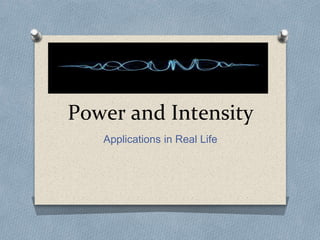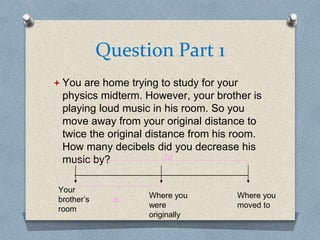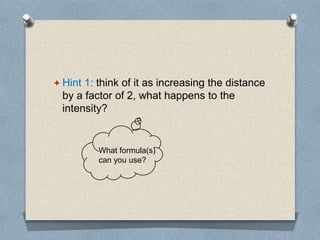Successfully reported this slideshow.

# Power and Intensity - LO4 Physics 101×

# Power and Intensity - LO4 Physics 101

A powerpoint presentation demonstrating two problems with solutions that explore applications of power and intensity in real life. It provides steps on how to derive equations to solve questions.

A powerpoint presentation demonstrating two problems with solutions that explore applications of power and intensity in real life. It provides steps on how to derive equations to solve questions.

### Power and Intensity - LO4 Physics 101

1. 1. Power and Intensity Applications in Real Life
2. 2. Question Part 1  You are home trying to study for your physics midterm. However, your brother is playing loud music in his room. So you move away from your original distance to twice the original distance from his room. How many decibels did you decrease his music by? Your brother’s room Where you were originally Where you moved to d 2d
3. 3.  Hint 1: think of it as increasing the distance by a factor of 2, what happens to the intensity? What formula(s) can you use?
4. 4.  Hint 1: think of it as increasing the distance by a factor of 2, what happens to the intensity?  Hint 2: How do you relate intensity to decibels?
5. 5. Solution to Question Part 1 O Initial (15-30) page 252 of textbook (15-28) page 251 of textbook
6. 6. Does your solution make sense?  This solution means that as you moved further away from his room, the intensity of his music decreased, and therefore the decibels you hear has decreased. So yes! This solution makes logical sense.
7. 7. Question Part 2  You go into your very quiet sound studio to get away from your brother’s music. Inside the studio, the pressure amplitude of sound waves is 30.0 μPa. What is the intensity of sound you hear in the studio? Is this sound detectable? (assuming its 20°C in your house)
8. 8. How to Approach These Types of Questions 1) When a question has too many unknowns, we can start by listing the values we do know, either from what the question tells us and/or set values in data tables. 2) We can then write down the main formula that will calculate what the question is ultimately asking for (in this case intensity). 3) Then, list out all the formulas that are related to the variables in the main formula. 4) Substitute in the formulas to possibly cancel out unknowns. 5) Plug in values and solve!
9. 9.  Hint 1: The bulk modulus, speed of sound, and density of air at 20 °C is given in Table 15-1 of the textbook Physics for Scientists and Engineers – An Interactive Approach
10. 10.  Hint 1: The bulk modulus, speed of sound, and density of air at 20 °C is given in Table 15-1 of the textbook Physics for Scientists and Engineers – An Interactive Approach  Hint 2: Think about how to cancel out frequency in the formula since it was not given in the question.
11. 11. Solution to Question Part 2 Continued Next Slide Main Formula (15-13) page 242 of textbook (15-11) page 241 of textbook (13-9) page 161 of textbook (15-11) page 241 of textbook
12. 12. Solutions to Question Part 2
13. 13. Does Your Solution Make Sense? • An intensity of 2.14 x 10^(-12) W/m^2 is BARELY noticeable to the human ear because it is still slightly higher than the lowest intensity detectable by the human ear, which is 10^(-12) W/m^2. As you can see, the two values are very similar. This is an amazing sound studio! 13
14. 14. Work Cited  Physics Textbook(for equations): Physics for scientist and Engineers – An Interactive Approach
15. 15. Thank You for Your Time!